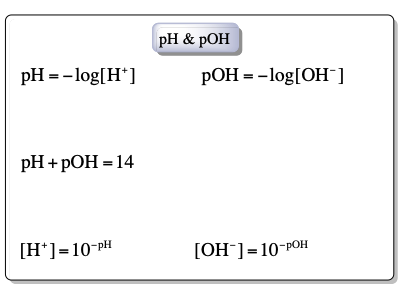Clutch Prep is now a part of Pearson
Ch.15 - Acid and Base EquilibriumWorksheetSee all chapters

# The pH Scale

See all sections
Sections
Acids Introduction
Bases Introduction
Binary Acids
Oxyacids
Bases
Amphoteric Species
Arrhenius Acids and Bases
Bronsted-Lowry Acids and Bases
Lewis Acids and Bases
The pH Scale
Auto-Ionization
Ka and Kb
pH of Strong Acids and Bases
Ionic Salts
pH of Weak Acids
pH of Weak Bases
Diprotic Acids and Bases
Diprotic Acids and Bases Calculations
Triprotic Acids and Bases
Triprotic Acids and Bases Calculations
Conjugate Acids and Bases

The pH scale is used to determine if a solution is acidic, basic or neutral.

###### pH Scale

Concept #1: Under standard conditions and concentrations less than 1.0 M, the pH scale ranges from 0 to 14.

Example #1: Given the [H+] of the following solutions, which one is the least acidic?

a) HNO3: [H+] = 1.2 M

b) HCl: [H+] = 0.025 M

c) H2SO4: [H+] = 0.27 M

d) HClO4: [H+] = 0.019 M

Concept #2: The concentrations of H+ and OH can help determine the pH and pOH of solutions respectively.

Example #2: A solution is prepared by dissolving HCN in 2 L of water. The [H+] was found to equal to 0.34 moles. Calculate the pH of this HCN solution.

Practice: A solution of NaOH was prepared in a chemistry lab and the pOH was determined to be 9.3. What is the concentration of OH ions of this basic solution?

Concept #3: Under standard conditions, the addition of pH and pOH is equal to 14.Example #3: You prepare a solution of HCl with a pH of 2.3 at 25ºC. What would be the pOH and the concentration of hydronium ions of this solution?

Practice: Calculate [OH] of a lemon juice solution at 25°C with a [H+] = 5.7 × 104 M.

Practice: A 345 mL bottle of antacid (Mg(OH)2) contains 1.45 × 10−2 moles of hydroxide ions. Determine pH and pOH of the antacid.

Practice: Which of the following statement(s) on aqueous solutions is/are correct?

a) aqueous solutions have a pH of 7

b) as concentration of hydronium ion increases, concentration of hydroxide ion decreases

c) solutions of weaker acids generally have a higher pOH then solutions of stronger acids

d) pH of pure water equals to 7 at 35º C.